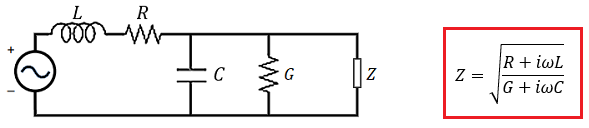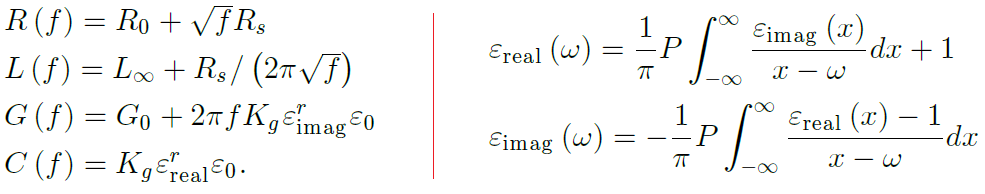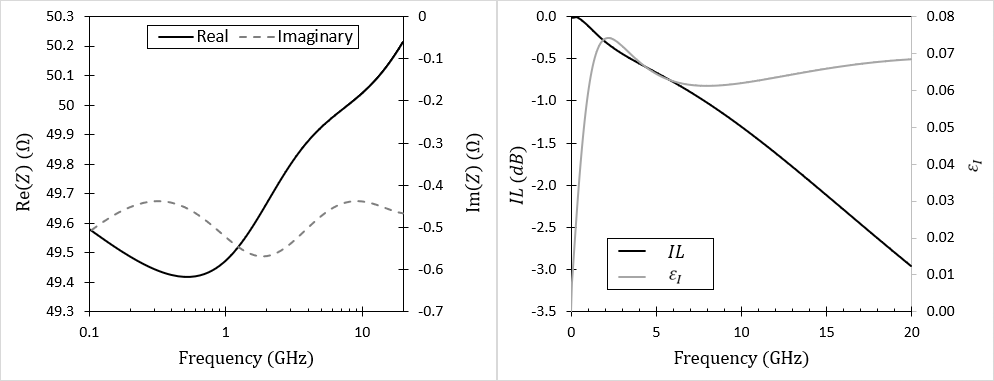# Dispersion and Losses in Transmission Line Characteristic ImpedanceRLCG(f) model for transmission line impedance without dispersion and skin effect losses.RLCG(f) model transmission line impedance approximation.
• C and G are functions of frequency. Both of these terms are functions of frequency as they depend on the dielectric constant (real and imaginary parts, respectively).
• The skin effect is ignored. The skin effect adds some resistance to the line in addition to the native DC resistance of the conductor. The skin effect also adds some reactive impedance to the line as it creates eddy magnetic fields, thus it adds a frequency-dependent contribution to L.(Left) Frequency-dependent parameters in the RLCG(f) model describing causal transmission lines, including transmission line dispersion, DC losses, dielectric losses, and the skin effect. (Right) Kramers-Kronig relations between the real and imaginary parts of the dielectric function.

# Effects of Dispersion and Losses on Impedance and Insertion Loss(Left) Characteristic impedance, and (Right) Insertion loss and imaginary part of the dielectric function in the designed stripline with optimized geometry.

# Managing Transmission Line Dispersion

• They don’t use experimental data for the dielectric function. Most designers will simply assume a specific value for dielectric constant, and they often do this at the incorrect frequency. While a single value for the dielectric constant might be fine for a harmonic signal, it’s simply wrong when working with a digital signal, especially at fast rise time.
• They don’t know how to calculate a transmission line’s impedance spectrum. I’ve never personally met a designer that knows how to do this correctly. However, I know some designers that understand the importance of calculating the impedance spectrum throughout the bandwidth; they usually do this using a finite-difference frequency-domain (FDFD) post-layout simulation. However, you can do this analytically without using field solvers.

--

--